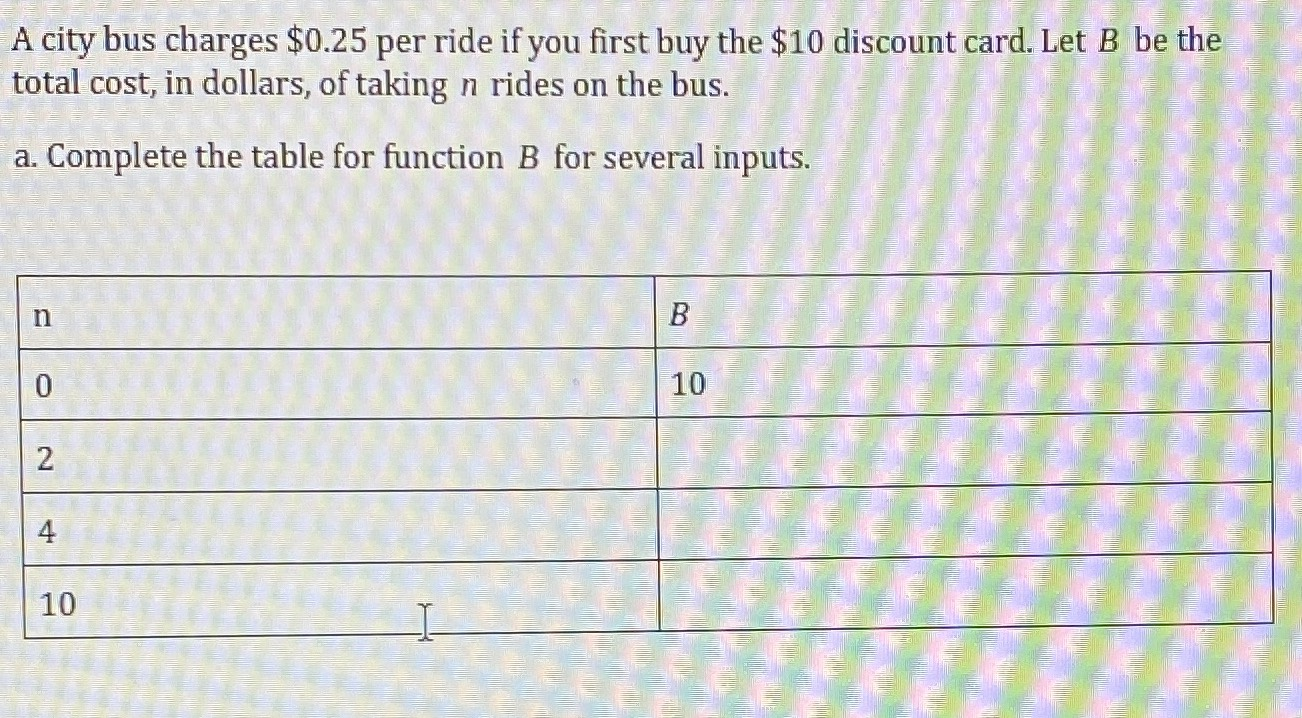### ¿Todavía tienes preguntas de matemáticas?

Pregunte a nuestros tutores expertos
Algebra
PreguntaA city bus charges $0.25 per ride if you first buy the $$\ 10$$ discount card. Let $$B$$ be the total cost, in dollars, of taking $$n$$ rides on the bus. a. Complete the table for function .25 per ride if you first buy..." crossorigin="anonymous" src="https://static.cameramath.com/jkyx9y2yhe/6cdbde26daa8216fb86620cf7cdd3f05" data-width="1302" data-height="718"> A city bus chargesA city bus charges$0.25 per ride if you first buy the $$\ 10$$ discount card. Let $$B$$ be the total cost, in dollars, of taking $$n$$ rides on the bus. a. Complete the table for function .25 per ride if you first buy the $$\ 10$$ discount card. Let $$B$$ be the total cost, in dollars, of taking $$n$$ rides on the bus. a. Complete the table for function $$B$$ for several inputs.